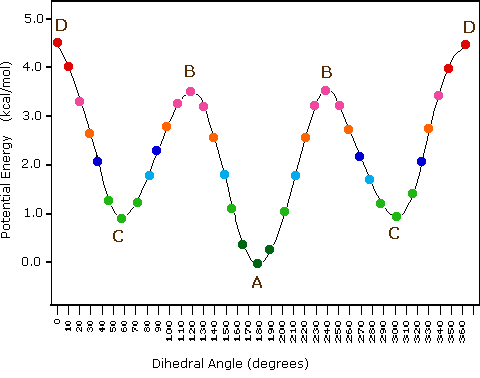# 3.4: Conformations of Butane

$$\newcommand{\vecs}{\overset { \rightharpoonup} {\mathbf{#1}} }$$ $$\newcommand{\vecd}{\overset{-\!-\!\rightharpoonup}{\vphantom{a}\smash {#1}}}$$$$\newcommand{\id}{\mathrm{id}}$$ $$\newcommand{\Span}{\mathrm{span}}$$ $$\newcommand{\kernel}{\mathrm{null}\,}$$ $$\newcommand{\range}{\mathrm{range}\,}$$ $$\newcommand{\RealPart}{\mathrm{Re}}$$ $$\newcommand{\ImaginaryPart}{\mathrm{Im}}$$ $$\newcommand{\Argument}{\mathrm{Arg}}$$ $$\newcommand{\norm}{\| #1 \|}$$ $$\newcommand{\inner}{\langle #1, #2 \rangle}$$ $$\newcommand{\Span}{\mathrm{span}}$$ $$\newcommand{\id}{\mathrm{id}}$$ $$\newcommand{\Span}{\mathrm{span}}$$ $$\newcommand{\kernel}{\mathrm{null}\,}$$ $$\newcommand{\range}{\mathrm{range}\,}$$ $$\newcommand{\RealPart}{\mathrm{Re}}$$ $$\newcommand{\ImaginaryPart}{\mathrm{Im}}$$ $$\newcommand{\Argument}{\mathrm{Arg}}$$ $$\newcommand{\norm}{\| #1 \|}$$ $$\newcommand{\inner}{\langle #1, #2 \rangle}$$ $$\newcommand{\Span}{\mathrm{span}}$$$$\newcommand{\AA}{\unicode[.8,0]{x212B}}$$

Learning Objective

• interpret and draw the rotation about a carbon-carbon single bond using Newman projections and sawhorse structures
• correlate energies of conformations with rotational energy diagrams and predict the most stable conformations for butane

Butane Conformations

Now let's consider butane, with its four-carbon chain. There are now three rotating carbon-carbon bonds to consider, but we will focus on the middle bond between C2 and C3. Below are two representations of butane in a conformation which puts the two CH3 groups (C1 and C4) in the eclipsed position, with the two C-C bonds at a 0o dihedral angle.If we rotate the front, (blue) carbon by 60° clockwise, the butane molecule is now in a staggered conformation.This is more specifically referred to as the gauche conformation of butane. Notice that although they are staggered, the two methyl groups are not as far apart as they could possibly be.

A further rotation of 60° gives us a second eclipsed conformation (B) in which both methyl groups are lined up with hydrogen atoms.One more 60 rotation produces another staggered conformation called the anti conformation, where the two methyl groups are positioned opposite each other (a dihedral angle of 180o).As with ethane, the staggered conformations of butane are energy 'valleys', and the eclipsed conformations are energy 'peaks'. However, in the case of butane there are two different valleys, and two different peaks. The gauche conformation is a higher energy valley than the anti conformation due to steric strain, which is the repulsive interaction caused by the two bulky methyl groups being forced too close together. Clearly, steric strain is lower in the anti conformation. In the same way, steric strain causes the eclipsed A conformation - where the two methyl groups are as close together as they can possibly be - to be higher in energy than the two eclipsed B conformations.

The diagram below summarizes the relative energies for the various eclipsed, staggered, and gauche conformations.The following diagram illustrates the change in potential energy that occurs with rotation about the C2–C3 bond at smaller rotational increments.Potential curve vs dihedral angle of the C2-C3 bond of butane.

Because the anti conformation (staggered) is lowest in energy (and also simply for ease of drawing), it is conventional to draw open-chain alkanes in a 'zigzag' form, which implies anti conformation at all carbon-carbon bonds. The figure below shows, as an example, a Newman projection looking down the C2-C3 bond of octane.Exercise

1: Using free rotation around C-C single bonds, show that (R,S) and (S,R)-tartaric acid are identical molecules.2: Draw a Newman projection, looking down the C2-C3 bond, of 1-butene in the conformation shown below (C2 should be your front carbon).Solutions to exercises